# True Random Number GenerationWritten by

## Using Comparators

### The top requirement of true random numbers is that they be products of pure chance—impossible to predict or reconstruct. Without countermeasures, a typical comparator will oscillate in unpredictable ways. In this project article, Wolfgang shares how he employed these oscillations to generate random numbers. The article describes the principles of operation together with its subtleties and “gotchas.”

• How to generator true random numbers using comparators

• How do comparators behave?

• How to shape the waveform

• How to leverage the oscillations from comparators

• How to set up the experiment

• How to analyze the oscillations more in depth

• How to understand the drops and spikes

• How to pick-up (collar) some contents of the oscillation counter

• Texas Instruments comparators (see RESOURCES)

• Maxim Integrated comparators (see RESOURCES)

• Analog Devices comparators (see RESOURCES)

The use of random numbers—for example, in encryption—has been going on for a long time. Here we will concentrate on a method to generate such numbers. As the most essential requirement, random numbers should be products of pure chance—like the numbers drawn in a lottery. Nobody should be able to predict or reconstruct such a number.

In terms of how they are generated, there are two kinds of random numbers: the pseudo- and the true-random numbers. Pseudo-random numbers are numerical values or bit patterns that do not appear to be regular at first glance but are generated by deterministic devices or algorithms. To reconstruct them requires only computing power and time—hopefully both beyond the capabilities of the bad guys. In contrast, true-random numbers (TRNs) are generated by physical processes showing an inherent random behavior. Because of this, the randomness comes from a physical process and not from a digital automaton, it is ideally not possible to reconstruct a random number once it has been generated—even if the same generating apparatus is used. All that said, it cannot be ruled out that random numbers being equal to one another are generated. However, the likelihood of that occurring is very low.

Various physical processes have been investigated and used to generate true random numbers. What we will discuss here can be thought of as an open-source design idea. We cannot deliver a blueprint of a ready-to-use random number generator, not to mention a certified one. Experiments have been conducted only to demonstrate the feasibility. The project status, the open-source license conditions and more details may be found in the accompanying material provided in RESOURCES at the end of this article.

HOW COMPARATORS BEHAVE
The comparator (Figure 1) is a high-gain, non-feedback differential amplifier. The purpose of a comparator is to compare the signal levels at the inputs with each other and to provide a binary comparison result. The output should assume only one of two levels (low or high), depending on the voltage difference VDIFF between the inputs. VDIFF = VIN+ – VIN–. If VIN– is greater than VIN+, VDIFF is negative, and the output is low. If VIN+ is greater than VIN–, VDIFF is positive, and the output is high. The comparator, however, has no built-in threshold or snap-action mechanism. The threshold effect results solely from the high voltage gain, causing even a small differential voltage VDIFF to overdrive the amplifier.Figure 1 – The typical comparator has five pins: a positive input, a negative input, an output and two pins to connect to the supply voltage. The output should behave like a switch. The output level depends on the voltage difference between the inputs. If this difference is positive, the comparator emits a high level. Otherwise, if the difference is negative, the output will assume a low level. This simple explanation leaves open the question of how the comparator will behave if the difference is zero.

If VIN+ is equal to VIN– or if VDIFF is very small, then no overdriving will occur, in spite of the large voltage gain. The comparator will behave erratically. The relevant parameter is the input offset voltage (VOS). Comparators have lower and upper limits of the offset voltage. The region between the two limits –VOS and +VOS is called the linear region (Figure 2).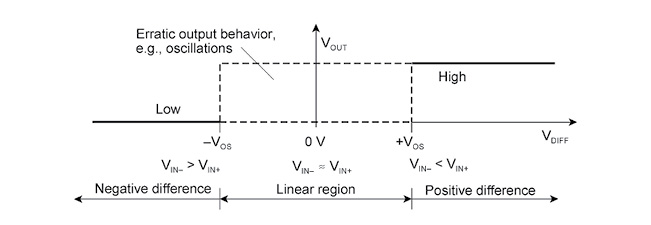FIGURE 2 – This shows how the output voltage VOUT depends on the difference VDIFF between the input voltages. In the linear region, the comparator’s output will behave erratically.

A definite output signal (low or high) can be expected only if the input differential voltage (VDIFF) is beyond the lower limit (-VOS) or above the upper limit (+VOS) of the input offset voltage. Figure 3 shows an input signal compared to a reference voltage. If the input differential voltage remains in the linear region for a somewhat longer time, the comparator’s output will oscillate (Figure 4a). On the other hand, if the linear region is traversed fast enough, the comparator will find no opportunity to switch back and forth. Hence, only a single signal edge will occur (Figure 4b). If the input differential voltage changes extremely slowly, it may occur that the linear region is not traversed, but crossed again in the opposite direction (Figure 4c). This can result in a variety of wild oscillations. Figure 5 shows some examples.FIGURE 3 – Shown here is the most basic application circuit. One comparator input is fed with a time-variable signal. At the other input, a reference voltage has been applied.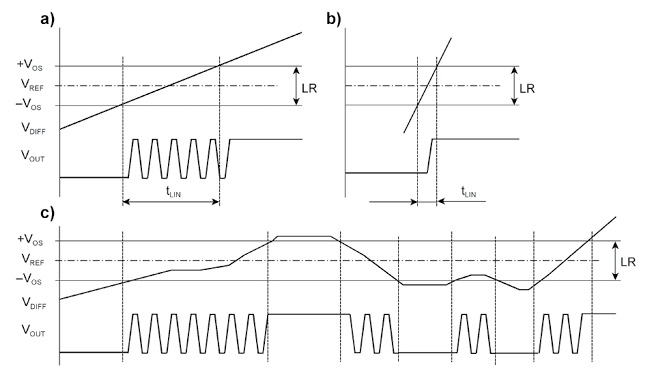FIGURE 4 – Whether the comparator oscillates or not depends on how long the input voltage difference VDIFF remains in the linear region LR (dwell time tLIN).

THE PRINCIPAL IDEA
When looking at diagrams as shown in Figure 4 and Figure 5, the idea is not that far away to make good use of such oscillations. Obviously, an oscillating comparator shows a somewhat random behavior. Perhaps, it can serve as a usable source of entropy. With the circuitry shown in Figure 3 as a starting point, it’s not difficult to build a random number generator (Figure 6).

The comparator compares a variable input voltage to a reference voltage. The input voltage is generated so that the input difference voltage remains in the linear region sufficiently long, causing the comparator to oscillate. The random number is produced by counting. The number or the width of the pulses or both can be used as an entropy source. The most straightforward approach is to connect the comparator’s output to the clock input of a counter and to interpret the counter’s content as a random number. The simplest use as an entropy source is to extract a single random bit when the input signal has traversed the linear region. Each pass corresponds to tossing a coin or rolling a die.

We will consider the least-significant counter bit the random bit. The decisive question is whether or not the counting produces sufficient randomness (entropy). To this end, we evaluate the complete counter content. For this, we have provided a counter and not simply a toggle flip-flop. The evaluation circuitry energizes a validity signal, indicating whether or not the random bit is considered valid. Moreover, the evaluation influences the signal generator too. It is some kind of adaptive regulation. Most of the oscillations should yield usable random bits. In order to achieve this goal, the shape of the input signal, its rise time, dwell time and so on will be adapted accordingly.

SHAPING THE WAVEFORM
The comparator should not oscillate if the time the differential input voltage traverses the linear region (tLIN) is shorter than the response time (datasheet value tRESP)—in other words:

This yields a slew rate (for example, in V/µs) of:

Here, however, we are intentionally causing oscillations. Therefore, the slew rate must be considerably less and the input differential voltage must remain in the linear region for a certain time. On the other hand, it must not settle there, because then the comparator would continuously oscillate. Later, we will explain why this is not the preferred mode of operation.

The simplest input waveform is a linear rise or fall, traversing the linear region in a certain dwell time tLINFigure 7 illustrates how the slew rate must be chosen to produce useful random bit patterns. Figure 7a shows a steep increase with extremely short dwell time. The linear region is traversed too quickly. The comparator does not oscillate, but shows only one output transition and hence no entropy at all. According to Figure 7b, the increase is not that steep. The comparator is operated in the linear region for some time. When entering, the comparator will begin to oscillate. The oscillations will cease when the linear region has been left. Such bursts of oscillations may yield a good source of entropy. Figure 7c shows an extremely flat increase with a long dwell time. The comparator emits continuous oscillations that are not that well-suited as an entropy source.

The question may arise why we prefer a time-variable input signal instead of simply applying input voltages yielding a differential voltage within the linear region. Then, the comparator would oscillate permanently, as shown in Figure 7c. This will not work, however, because the oscillations occur in a narrow region of the input differential voltage, depending on the comparator itself, on the temperature, the operating voltage and so on. It is a question of millivolts. So that the oscillations never cease, the differential voltage would have to be corrected again and again. To avoid this problem, we traverse the entire linear region cyclically. This way we will always detect the part of the linear region in which the comparator will oscillate.

Figure 8 and Figure 9 illustrate a basic algorithm to obtain random bits. To approach the region in which the comparator will oscillate, we begin at an input voltage well beneath the reference voltage (VIN < VREF). VIN will be incremented in comparatively large steps. In each step, after a certain dwell time has elapsed, the content of the counter will be read in. When the first change is detected, a second loop will traverse the linear region in smaller steps with a somewhat longer dwell time. The loop ends when the input voltage is well above the reference voltage (VIN > VREF). Then the content of the counter will be read in again. If the count value exceeds a certain limit, we assume a sufficient number of oscillations has occurred. Then the lowest-order bit contributes to the random number. Otherwise, an error message will be emitted.FIGURE 8 – The input voltage should dwell within the linear region for some time, thus inciting a sufficient number of oscillations. On the other hand, it should not take too much time to reach this region. Therefore, we begin with a higher slew rate until the first oscillations are detected.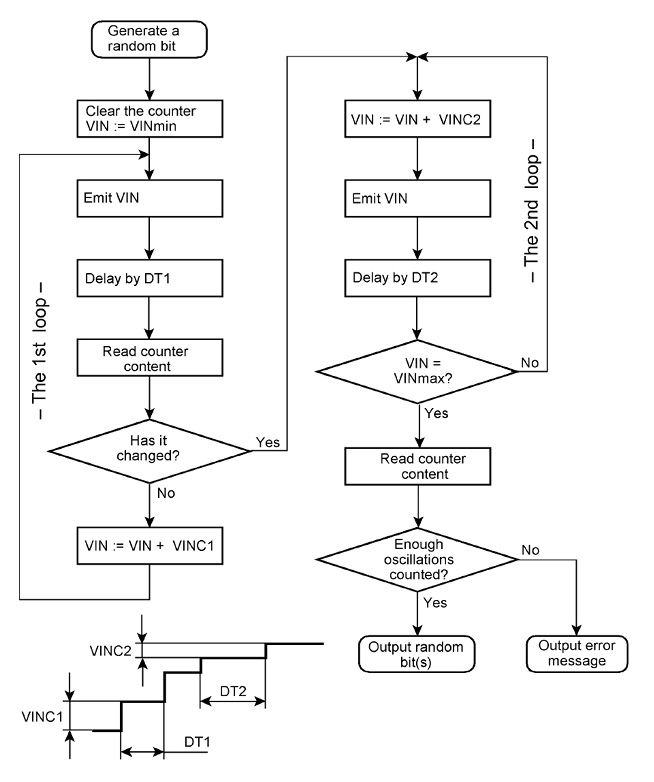FIGURE 9 – This flowchart depicts the basic algorithm. It can be implemented by a program or a state machine. Subtleties, like checking for errors, have been omitted.

THE FIRST EXPERIMENTAL SETUP
It goes without saying that it’s best to base an experimental device on a state-of-the-art microcontroller (MCU). Figure 10 shows a block diagram of an experimental platform, and Figure 11 shows a photo of it. The MCU’s digital-to-analog converter (DAC) generates the input signal. The comparator is an industry-standard IC, the counter and the evaluation circuitry have been implemented in a CPLD.FIGURE 10 – Block diagram of the first experimental setup. This machine delivers the bit patterns to an attached personal computer executing the more complex configuration and evaluation software.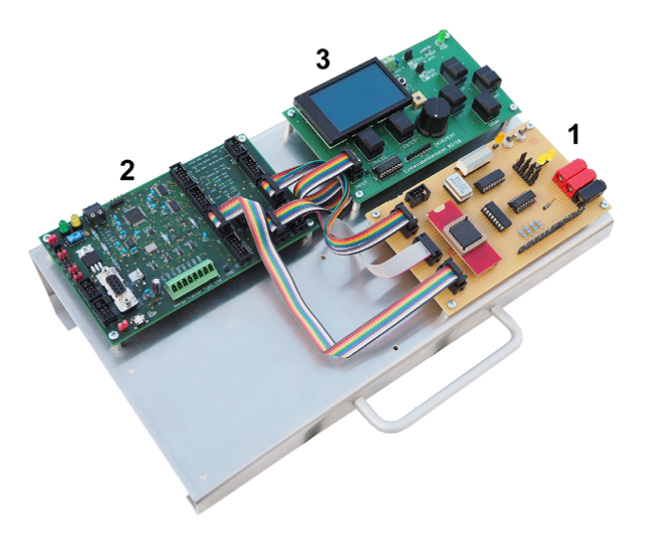FIGURE 11 – The experimental platform is a quick-and-dirty solution, built from available modules and cheap components. The decisive function blocks are an MCU with DACs and the prototyping board (1) with the comparators and a CPLD containing the digital circuitry. The other features of the MCU module (2) are not used. The human interface module (3) has been added to provide for tinkering without a personal computer attached.

The most demanding problem is the evaluation of the bit patterns. The first programs run on the experimental machine already showed impressive strings of ones and zeros. But are they truly random or not? Typically, professional certification suites will not be readily available. Besides, such programs need vast amounts of data, making experiments a time-consuming process. As a makeshift solution, I use a simple pre-evaluation algorithm based on common-sense rules.

It is easy to understand that, in a truly random bit string, the numbers of ones and zeros should be equal. This is also true for repetitive bit patterns—the so-called runs. There should be as many 11-patterns as 00-patterns, and as many 111-patterns as 000-patterns and so on. Assume, for example, two counters: one for the zeros, the other for the ones. Each new bit will advance the corresponding counter, while the other counter will be left idle. The difference between both count values is called bias. Similarly, the runs can be counted too.

During random number generation, the software examines whether the bias is increasing monotonously in one direction or not. A good random number generation process must result in a bias that does not always grow, but also decreases and changes its sign temporarily (sometimes more zeros than ones will appear, sometimes the opposite is true). A bit string will be accepted as a good random number only if the bias does not exceed a certain limit and does not grow monotonously.

My first experiments, however, have shown disappointingly low randomness. Occasionally, it could be observed even on the LCD display (shown in Figure 11) that certain bit patterns occur again and again. By tinkering with the signal parameters (recalibration), it was possible to generate bit strings with sufficiently low bias. With such parameter values, however, the machine did not work well over a longer period of time. Rather, it had to be recalibrated again and again. Searching for usable parameter values is a kind of a multi-dimensional optimization—where all parameters influence each other. With that mind, it took a comparatively long time to generate usable random bit strings.

OSCILLATIONS: A CLOSER LOOK
These observations led to my desire to investigate the oscillating behavior in more detail. At first glance, oscillations, as shown in Figure 5, seem to be chaotic. So, it is quite natural to expect they will yield good random numbers. Why, however, do the experiments not show the desired randomness?

The main cause of the oscillations is capacitive feedback from the output to the inputs. When the input difference voltage enters the linear region, the comparator will begin to switch. In the beginning, it is some kind of analog amplifier. A small increase or decrease of the input difference voltage will cause the output voltage to rise or fall, respectively. The change at the output is capacitively fed back to the inputs, therefore temporarily changing the differential voltage. If the input differential voltage increases, the output voltage can rise even more steeply. If it decreases, it can fall back to the initial value. These changes at the output are in turn fed back to the inputs. The comparator’s behavior can be observed in more detail by adding a capacitor, thereby providing for strong capacitive feedback (Figure 12).

Our experiments begin with a positive input voltage significantly lower than the negative input voltage. Consequently, the output voltage is low. The positive input voltage will be gradually increased until the input differential voltage enters the linear region. Then the input voltage is held constant. Here the comparator acts as an analog amplifier. Its behavior depends only on the capacitive feedback. The capacitor acts basically as a differentiator—edges will result in spikes or needles. An ascending edge will cause a positive spike, a descending edge a negative one.

When the input differential voltage enters the linear region, the comparator’s amplifier stages will cause the output voltage to rise. If, as shown in Figure 12a, that input voltage is fed back to the positive input, the spike will temporarily increase the differential voltage, causing the output voltage to increase even more. When the rise is comparatively slow, the feedback capacitor has enough time to discharge. The differential voltage drops and the output will fall back to the low level. On the other hand, if the output voltage rises more steeply, the capacitor is recharged, the differential voltage increases and the output level will rise according to an exponential function.

The output pulses either have a small amplitude or can reach a high level (Figure 13). The right part of the picture shows in detail how one of the small impulses is formed. In the input voltage trace (VIN+), the spikes can be seen. The spike (1) results from the initial increase (2) of the output voltage. It will cause an increase in the differential voltage and therefore a further ramp-up (3) of the output voltage level. When the spike has been faded away, the capacitor is discharged, the differential voltage decreases and the output voltage drops again (4). This results in a negative spike (5), further lowering the differential voltage. As a result, the comparator output remains at the low level for a certain time (6), until the capacitor has discharged again. Therefore, the intervals between the output pulses are comparatively wide.FIGURE 13 – These pulses may occur when the output is capacitively fed back to the positive input.

If, as shown in Figure 12b, the rise in the output voltage is fed back to the negative input, the differential voltage will reduce. As a result, the output level will fall. This drop is fed back to the input as a negative spike, thereby increasing the differential voltage, resulting in a higher voltage swing at the output. The rise is fed back as a positive spike reducing the differential voltage. Because the output follows the differential voltage, a falling edge will occur that is fed back to the input as a negative spike. This in turn will increase the differential voltage, which again causes the output voltage to rise and so on.

DROPS AND SPIKES
With the output level rising, the positive spikes will at some point reach such an amplitude that the voltage at the negative input is higher than at the positive. As a result, the output voltage will drop. These processes take place extremely quickly—every output change immediately has an opposite effect at the input (that’s principle of the feedback op amp). A differential amplifier with capacitive feedback to the negative input is, in principle, an integrator. It will deliver output pulses with a gently rising leading edge, which may be overlaid by high-frequency oscillations (self-excitation).

Such pulses are shown in Figure 14. The comparator emits oscillations with a comparatively low amplitude and high frequency. The right part of the picture shows an oscillation in detail. The spike (1) results from the initial increase (2) of the output voltage. According to the principle of an integrating amplifier, the feedback causes the output voltage to ascend steadily (3) until the input difference voltage reverses its polarity. The output voltage will drop (4), causing a negative spike (5), reversing the polarity of the differential voltage again. Thus, a new cycle begins (6). The details of the feedback processes cannot be observed, since their delay time is not determined by time constants of the capacitive feedback, but only by the propagation delay of the comparator. Therefore, the capacitive feedback may cause oscillations of extremely high frequencies, of which only the envelope can be observed.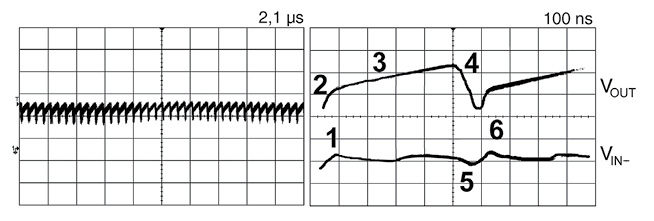FIGURE 14 – Pulses such as these may occur when the output is capacitively fed back to the negative input.

Figure 15Figure 16 and Figure 17 show oscillations emitted in cycles according to Figure 8. Without additional feedback, most of the pulses have steep edges and a full voltage swing between low and high (Figure 15). Capacitive feedback to the positive input causes spikes or sawtooth pulses with a low amplitude or pulses whose leading edges ascend to a high level according to an exponential function (Figure 16). Capacitive feedback to the negative input results in only a few spikes and an output voltage gradually increasing to the high level, overlaid by high-frequency (HF) oscillation phenomena (Figure 17).

In our experiments, the entire linear region is traversed, and all oscillations are counted. The explanations of the comparator’s behavior let us understand why this principle results in a source of entropy that may be usable, but is not really satisfying.

The input voltage follows the same time-function in each cycle. The interval the comparator is operated in the linear region remains practically the same in all cycles. Within short cycle times (milliseconds and below), the temperature remains practically the same. This means that the parameters which govern the feedback effects exhibit no significant randomness. If the input signal, reference voltage, cycle time, temperature and operating voltages are always the same, it is highly probable that the number of oscillations will be also the same.

All that said, such considerations are purely theoretical. Nothing is really constant. Rather, there are random processes that influence the onset of the oscillations and their duration. Figure 18 illustrates that the differences are in the details. Some impulses will be a little wider, some a little narrower, the oscillations will start a little earlier in one pass, a little later in the next and so on. This could be a useful source of entropy.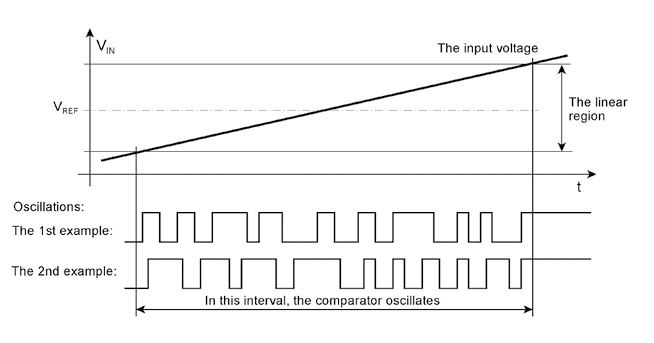FIGURE 18 – When voltage, timing and thermal parameters are nearly constant, entropy manifests itself in small variations of the comparator’s output.

MODIFIED METHOD, FOUND EMPIRICALLY
While the linear region is traversed, the counter will count and hence accumulate the number of oscillations. At certain time intervals, its content will be sampled and stored. These sampled values we refer to as collared values in order to avoid confusion with the usual technical term of sampling in analog-to-digital conversion and signal processing. The aim of sampling is to obtain values representing the original signal as true as possible. This is exactly what we want to avoid here, because a precise reconstruction of the original signal would also result in the original entropy. In contrast, we want to obtain samples showing pronounced randomness.

Figure 19 illustrates how collared values are stored. In practice, we have not built any such circuitry but implemented the collaring and evaluation by software. The memory is simply an area in the MCU’s RAM, and the address counter resides in a register. The input voltage waveform is generated as shown in Figure 8 and Figure 9. While the input voltage traverses the linear region, counter values will be stored. The collaring routine will be initiated by interrupts or called cyclically in the 2nd loop (for comparison, see Figure 8 and Figure 9). Collaring pulses to trigger the interrupts may be generated by a counter/timer unit of the MCU or by an external pulse generator.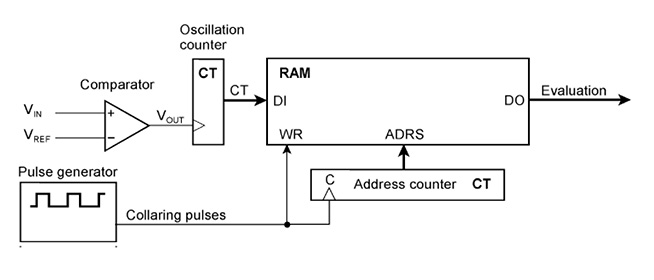FIGURE 19 – This block diagram visualizes the principle of picking up (collaring) some contents of the oscillation counter and storing them for further evaluation. In reality, capturing, storing and evaluating the counter values has been implemented by software.

After the linear region has been traversed, the collared values will be evaluated by software. A simple algorithm would be to add these values to one another and use the least significant bit of the sum as the random bit. However, bit strings generated this way, show still considerable bias. Therefore, the values contributing to the random bit are selected from the collared values according to further criteria. Experiments have shown that the entropy will improve considerably when that criteria are met. Figure 20 illustrates the principal idea behind it.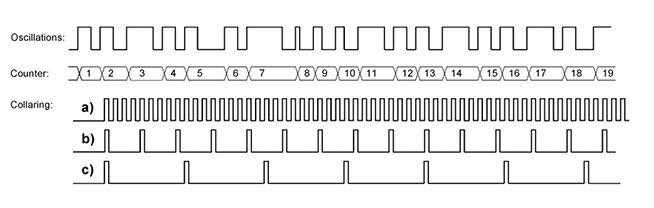FIGURE 20 – Counting oscillations and collaring the counter values. (a), (b) and (c) show collaring pulses at different intervals.

In case (a) (as shown in Figure 20), each of the counter values is collared several times—there are more collared values than counter values. It is easy to see that summing up the same values will not increase the entropy. In case (b), the vast majority of counter values are collared at least once. Collared and counter values correspond approximately to one another. Practically, this will yield no entropy at all. In case (c), the counter values are collared only occasionally, with some of the counter values being skipped. Only such values may be considered to produce random bits.

The first criterion is to consider only values different from the preceding values. With that in mind, we exclude multiple values collared from the same counter content, as shown in Figure 20a. The second criterion evaluates the difference between consecutive values—current value minus preceding value. Consecutive values shall not have the same difference. For example, if the values 4, 7, 10 have been read, the 10 will be rejected because the difference between 10 and 7 is the same as between 7 and 4. This excludes values collared from consecutive counts (as shown in Figure 20b) or from multiple counts having equal distance.

The third criterion is that there must be a certain minimum number of values that have been accepted according to the first and second criteria. Only then a random bit will be generated. If the criteria are not met, the program emits an error code instead of a random bit. In our experiments, the evaluation is done by software. Alternatively, it can be done on the fly—in other words, without the need to store the collared values in a RAM—by appropriate circuitry.

SUMMARY AND SUGGESTIONS
Comparators oscillate if the input difference voltage remains within the linear region for some time. On the oscilloscope, we see bursts of erratic pulses. Maybe they are a useful source of entropy to generate TRNs. The method seems not that complicated. An input signal is generated so that the linear region is traversed cyclically. The oscillations are counted and the least significant bit or bits contribute to the desired bit string constituting the random number. Experiments have shown that the principle is feasible. That said, the simple algorithm yields a disappointingly low entropy, requiring repeated recalibrations.

Further thoughts and experiments have led me to a modified method. While the input signal traverses the linear region, the counter content is sampled (“collared”) multiple times. All the collared values are evaluated to recognize whether or not the comparator’s output exhibits sufficient randomness. Further experiments have shown promising results.

What I’ve described in this article are demonstrations of feasibility or proof of the concept, conducted with an improvised experimental setup and with intentional disregard for speed. This may serve as a starting point for creating thoroughly engineered solutions based on 32-bit MCUs and perhaps even FPGAs. Such development could be aimed, for example, at a device packaged in a USB stick. The speed problem could be solved by shortening the cycle time and by operating some or even many comparators in parallel.

Go to the Circuit Cellar article materials webpage for an extensive addendum to this article with project history, 23 supplemental Figures and other resources.RESOURCES

Reference designs and application notes:

Analog designers usually strife to avoid oscillations. The most common method is to provide for hysteresis, that is, to modify the reference voltage if the comparator’s output level has changed.
This is to avoid that the differential voltage at the inputs remains in the linear region for some time. As soon as the output switches, the reference voltage is changed so that a larger input voltage swing in the opposite direction is required to switch back again. If the output signal switches on, the reference voltage is reduced, if it switches off, it is increased again. So small variations of the input voltage cannot cause the output switching back, thus avoiding oscillations.

These papers and articles discuss the causes of the oscillations and how to prevent them. When reading with the converse intention, perhaps we could get some ideas on how to incite them

1. Kay, Art; Claycomb, Timothy: Comparator with Hysteresis Reference Design. TIDU020A. Texas Instruments Incorporated, 2013–2014.
https://www.ti.com/lit/ug/tidu020a/tidu020a.pdf

2. Guide to Adding Extra Hysteresis to Comparators. Application Note 3616. Maxim Integrated Products,  2005.
https://www.maximintegrated.com/en/design/technical-documents/app-notes/3/3616.html

3. Application Note 74. LM139/LM239/LM339 A Quad of Independently Functioning Comparators. Application Report SNOA654A. Texas Instruments, Incorporated, 2004–2013.
https://www.ti.com/lit/an/snoa654a/snoa654a.pdf

4. Grohe, Paul: Application Design Guidelines for LM339, LM393, TL331Family Comparators Including the New B-versions. Application Report SNOAA35A. Texas Instruments, Incorporated, 2019–2020.
https://www.ti.com/lit/an/snoaa35a/snoaa35a.pdf

Some data sheets:
5. LM339, LM239, LM139, LM2901 Quad Differential Comparators. Data Sheet SLCS006U. Texas Instruments, Incorporated, 1979–2018.

6. TLV4011 Precision Comparator with Integrated Reference. Data Sheet SLVSFL9.  Texas Instruments, Incorporated, 2020.

7. LMV727x Single and Dual, 1.8-V Low Power Comparators With Rail-to-Rail Input. Data Sheet  SNOSA56I.   Texas Instruments, Incorporated, 2003–2015.

8. MAX40002–MAX40005/ MAX40012–MAX40015 nanoPower 4-Bump Comparator in Ultra-Tiny 0.73mm x 0.73mm WLP/SOT23 Packages. Data Sheet 19-8574. Maxim Integrated Products, Inc., 2020.
https://datasheets.maximintegrated.com/en/ds/MAX40002-MAX40005.pdf

9. LTC1841/LTC1842/LTC1843 Ultralow Power Dual Comparators with Reference. Data Sheet 1284123f. Linear Technology Corporation, 1998 / Analog Devices, Inc., 2020.
https://www.analog.com/media/en/technical-documentation/data-sheets/184123f.pdf

We need comparators without built-in hysteresis. This obvious requirement could comparators in microcontrollers or FPGAs render not suitable. To be reasonable fast, the random number generator requires some or even many comparators. So especially tiny comparators are of particular interest.

10. Matthes, Wolfgang: Microcontroller Modules for the Ambitious. Circuit Cellar, Issue 312, July 2016

PUBLISHED IN CIRCUIT CELLAR MAGAZINE • DECEMBER 2020 #365 – Get a PDF of the issue

 Keep up-to-date with our FREE Weekly Newsletter! Don't miss out on upcoming issues of Circuit Cellar. Subscribe to Circuit Cellar Magazine Note: We’ve made the May 2020 issue of Circuit Cellar available as a free sample issue. In it, you’ll find a rich variety of the kinds of articles and information that exemplify a typical issue of the current magazine. Would you like to write for Circuit Cellar? We are always accepting articles/posts from the technical community. Get in touch with us and let's discuss your ideas.